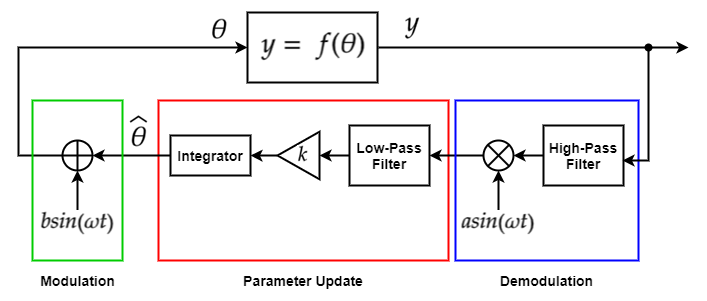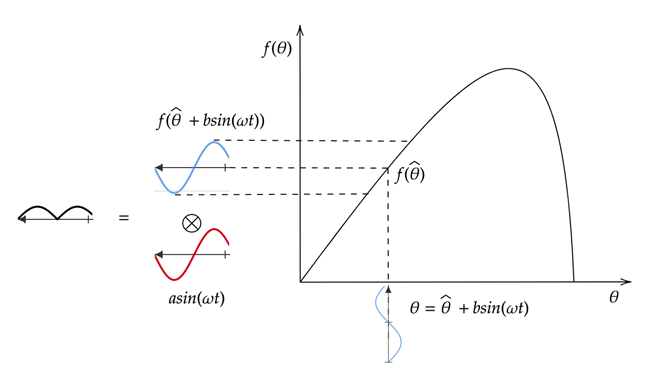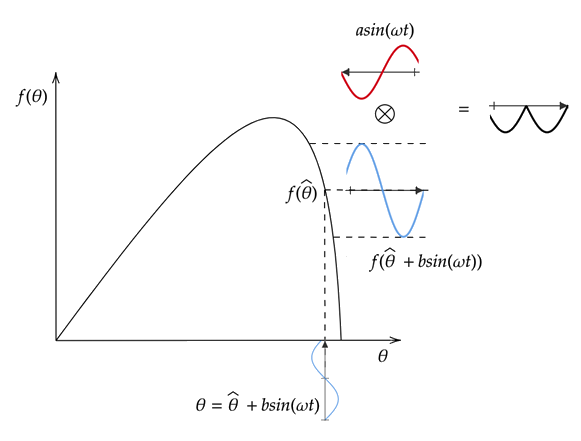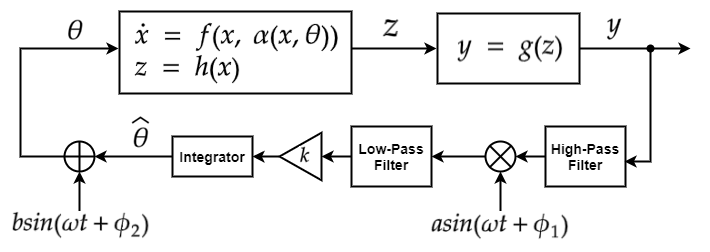Extremum Seeking Control

Extremum seeking control (ESC) is a model-free, real-time adaptive control algorithm that is useful for adapting parameters to unknown system dynamics and unknown mappings from control parameters to an objective function. You can use extremum seeking to solve static optimization problems and to optimize parameters of dynamic systems.

The extremum seeking algorithm uses the following stages to tune a parameter value.

1. Modulation — Perturb the value of the parameter being optimized using a low-amplitude sinusoidal signal.

2. System Response — The system being optimized reacts to the parameter perturbations. This reaction causes a corresponding change in the objective function value.

3. Demodulation — Multiply the objective function signal by a sinusoid with the same frequency as the modulation signal. This stage includes an optional high-pass filter to remove bias from the objective function signal.

4. Parameter Update — Update the parameter value by integrating the demodulated signal. The parameter value corresponds to the state of the integrator. This stage includes an optional low-pass filter to remove high-frequency noise from the demodulated signal.

Simulink® Control Design™ software implements this algorithm using the Extremum Seeking Control block. For examples of extremum-seeking control, see:

Time Domain

Using the Extremum Seeking Control block, you can implement both continuous-time and discrete-time controllers. The ESC algorithm is the same in both cases. Changing the time-domain of the controller affects the time domain of the high-pass filters, low-pass filters, and integrators used in the tuning loops.

To generate hardware-deployable code for the Extremum Seeking Control block, use a discrete-time controller.

The following table shows the continuous-time and discrete-time transfer functions for the filters and integrators in the Extremum Seeking Control block.

Controller ElementContinuous-Time Transfer FunctionDiscrete-Time Transfer Function
High-pass filter

$\frac{s}{s+{\omega }_{h}}$

$\frac{1-{z}^{-1}}{1-{\omega }_{h}{z}^{-1}}$

Low-pass Filter

$\frac{1}{s+{\omega }_{l}}$

$\frac{1}{1-{\omega }_{l}{z}^{-1}}$

Integrator

$\frac{1}{s}$

Forward Euler:

$\frac{{T}_{s}}{z-1}$

Backward Euler:

$\frac{{T}_{s}z}{z-1}$

Trapezoidal:

$\frac{{T}_{s}}{2}\frac{z+1}{z-1}$

Here:

• ωl is the low-pass filter cutoff frequency.

• ωh is the high-pass filter cutoff frequency.

• Ts is the sample time of the discrete-time controller learning rate.

Static Optimization

To demonstrate extremum seeking, consider the following static optimization problem.Here:

• $\stackrel{^}{\theta }$ is the estimated parameter value.

• θ is the modulation signal

• y = f(θ) is the function output being maximized, that is, the objective function.

• ω is the forcing frequency of the modulation and demodulation signals.

• b·sin(ωt) is the modulation signal.

• a·sin(ωt) is the demodulation signal.

• k is the learning rate.

The optimum parameter value, θ*, occurs at the maximum value of f(θ).

To optimize multiple parameters, you use a separate tuning loop for each parameter.

The following figure demonstrates extremum seeking for an increasing portion of the objective function curve. The modulated signal θ is the sum of the current estimated parameter and the modulation signal. Applying f(θ) produces a perturbed objective function with the same phase as the modulation signal. Multiplying the perturbed objective function by the demodulation signal produces a positive signal. Integrating this signal increases the value of θ, which moves it closer to the peak of the objective function.The following figure demonstrates extremum seeking for a decreasing portion of the objective function curve. In this case, applying f(θ) produces a perturbed objective function that is 180 degrees out of phase from the modulation signal. Multiplying by the demodulation signal produces a negative signal. Integrating this signal decreases the value of θ, which moves it closer to the peak of the objective function.The following figure demonstrates extremum seeking for a flat portion of the objective function curve, that is, a portion of the curve near the maximum. In this case, applying f(θ) produces a near-zero perturbed objective function. Multiplying by the demodulation signal and integrating this signal does not significantly change the value of θ, which is already near its optimum value θ*.Dynamic System Optimization

Extremum seeking optimization of a dynamic system occurs in a similar fashion as static optimization. However, in this case, the parameter θ affects the output of a time-dependent dynamic system. The objective function to be maximized is computed from the system output. The following figure shows the general tuning loop for a dynamic system.Here:

• $\stackrel{˙}{x}=f\left(x,\alpha \left(x,\theta \right)\right)$ is the state function of the dynamic system.

• z = h(x) is the output of the dynamic system.

• y = g(z) is the objective function derived from the output of the dynamic system.

• ϕ1 is the phase of the demodulation signal.

• ϕ2 is the phase of the modulation signal.

ESC Design Guidelines

When designing an extremum-seeking controller, consider the following guidelines.

• Ensure that the system dynamics are on the fastest time scale, the forcing frequencies are on the medium time scale, and the filter cutoff frequencies are on the slowest time scale.

• Specify an amplitude for the demodulation signal that is much greater than the modulation signal amplitude (ab).

• Select phase angles for the modulation and demodulation signals such that cos(ϕ1ϕ2) > 0.

• When tuning multiple parameters, the forcing frequency for each tuning loop must be different.

• Try designing your system without high-pass and low-pass filters. If the performance is not satisfactory, you can then consider adding one or both filters.

 Ariyur, Kartik B., and Miroslav Krstić. Real Time Optimization by Extremum Seeking Control. Hoboken, NJ: Wiley Interscience, 2003.